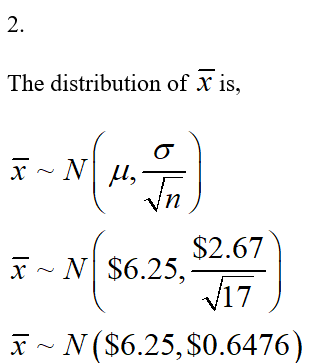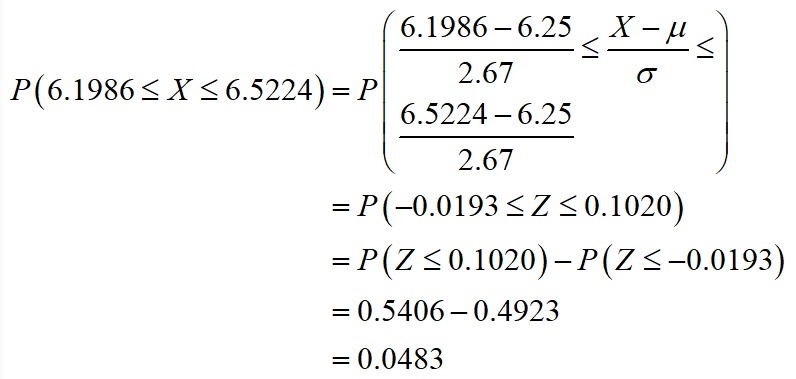# The average amount of money spent for lunch per person in the college cafeteria is \$6.25 and the standard deviation is \$2.67. Suppose that 17 randomly selected lunch patrons are observed. Assume the distribution of money spent is normal, and round all answers to 4 decimal places where possible.What is the distribution of XX? XX ~ N(,)What is the distribution of ¯xx¯? ¯xx¯ ~ N(,)For a single randomly selected lunch patron, find the probability that this patron's lunch cost is between \$6.1986 and \$6.5224. For the group of 17 patrons, find the probability that the average lunch cost is between \$6.1986 and \$6.5224. For part d), is the assumption that the distribution is normal necessary?

Question
1 views

The average amount of money spent for lunch per person in the college cafeteria is \$6.25 and the standard deviation is \$2.67. Suppose that 17 randomly selected lunch patrons are observed. Assume the distribution of money spent is normal, and round all answers to 4 decimal places where possible.

1. What is the distribution of XX? XX ~ N(,)
2. What is the distribution of ¯xx¯? ¯xx¯ ~ N(,)
3. For a single randomly selected lunch patron, find the probability that this patron's lunch cost is between \$6.1986 and \$6.5224.
4. For the group of 17 patrons, find the probability that the average lunch cost is between \$6.1986 and \$6.5224.
5. For part d), is the assumption that the distribution is normal necessary?
check_circle

Step 1

1.

The distribution of X is, X~N(\$6.25, \$2.67).

Step 2Step 3

3.

The probability that one randomly selected patron lunch cost between \$6.1986 and \$6.5224 is,Thus ,the probability that one randomly selected patron lunch cost between \$6.1986 and \$6.5224 is 0.0483.

...

### Want to see the full answer?

See Solution

#### Want to see this answer and more?

Solutions are written by subject experts who are available 24/7. Questions are typically answered within 1 hour.*

See Solution
*Response times may vary by subject and question.
Tagged in